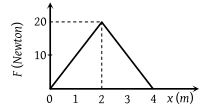The graph between the resistive force F acting on a body and the distance covered by the body is shown in the figure. The mass of the body is 25 kg and initial velocity is 2 m/s. When the distance covered by the body is 4m, its kinetic energy would be(1) 50 J

(2) 40 J

(3) 20 J

(4) 10 J

Concept Videos :-

#3 | Work Done by Variable Force

Concept Questions :-

Work done by variable force

(4) Initial K.E. of the body = $\frac{1}{2}m{v}^{2}=\frac{1}{2}×25×4=50\text{\hspace{0.17em}\hspace{0.17em}}J$

Work done against resistive force

= Area between F-x graph

= $\frac{1}{2}×4×20=40J$

Final K.E. = Initial K.E. – Work done against resistive force

= 50 – 40 = 10 J

Difficulty Level:

• 13%
• 31%
• 11%
• 48%